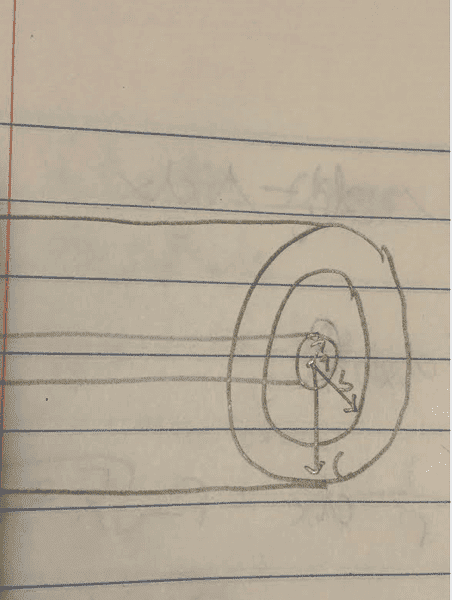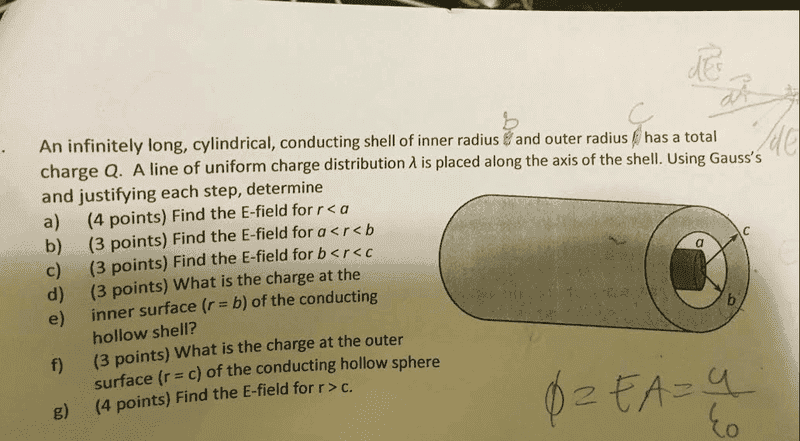# Gaussian surface (infinitely long cylindrical conductor)

## Homework Statement

An infinitely long, cylindrical, conducting shell of inner radius b and outer radius c has a total charge Q. A line of uniform charge distribution Λ is placed along the axis of the shell. Using Gauss's Law and justifying each step, determine. A) The Electric Field for r>a, B)The Electric Field for a<r<b, C) Find the Electric Field for b<r<c D) What is the charge at the inner surface ( r = b ) of the conducting hollow shell? E) What is the charge at the outer surface (r=c) of the conducting hollow sphere? F) Find the Electric Field for r > c

2. Homework Equations

∫EdA = qinside
Λ = Q/L
Q = λL
A = 2πrL

3. The Attempt at a Solution

So far I have gotten part B, C and D correct however I'm having trouble on parts A, E and F.
For part B i was able to get, E = Q/2πrLε, For Part C I know that the field inside of the conducting shell will be 0. And for part D I realize that the charge at r = b which already has a charge of Q inside will have a -Q charge in order for the total charge inside the conducting shell to be zero. For part A I got E = Λ/2πrε. Part E I thought that the charge with r = c would be Q - Q = qinside and for part F I substituted this value in to get E = Q-Q/2πrLε. Please Help?

Last edited:

TSny
Homework Helper
Gold Member
Hello, Kaleem.

The wording of this problem is odd. If you put a finite amount of charge Q on conducting cylindrical shell of infinite length, the charge would spread out such that the effect of Q would be negligible.

The problem refers to some distance labeled "a", but there is no explanation of what this distance "a" represents.
Part (a) wants the field for r > a and part (b) wants the field for a < r < b. But the regions r > a and a < r < b overlap each other.

You use the symbol "Q" to mean two different things: first, it represents the amount of charge on the cylindrical shell and then it represents some charge on the axis where you write Λ = Q/L. What particular length does L represent here?

Did you write the statement of the problem exactly as given to you?

Hello, Kaleem.

The wording of this problem is odd. If you put a finite amount of charge Q on conducting cylindrical shell of infinite length, the charge would spread out such that the effect of Q would be negligible.

The problem refers to some distance labeled "a", but there is no explanation of what this distance "a" represents.
Part (a) wants the field for r > a and part (b) wants the field for a < r < b. But the regions r > a and a < r < b overlap each other.

You use the symbol "Q" to mean two different things: first, it represents the amount of charge on the cylindrical shell and then it represents some charge on the wire where you write Λ = Q/L. What particular length does L represent here?

Did you write the statement of the problem exactly as given to you?

I made one mistake in which part A) is suppose to be r<a rather than r>a, sorry about that. Other than that everything else is written exactly, like how the problem stated it. I also believe L is suppose to represent the length of the cylinderical shell.

TSny
Homework Helper
Gold Member
I guess we should think of the cylinder as being "very long" (of length L) rather than infinitely long.

I think the only reasonable interpretation of the distance "a" is that it represents the radius of the charge distribution along the axis of the cylinder. So, this charge along the axis has a volume distribution. But then I would think that Λ probably refers to the volume charge density of the axial charge rather than the linear charge density. You can see the amount of guessing we need to make just to interpret the problem.

If this interpretation is correct, then part (a) is asking for E inside the volume charge distribution along the axis. You will need to introduce a Gaussian surface that would be appropriate for this region. Can you offer a suggestion for the geometrical shape that you would choose for the Gaussian surface?

I guess we should think of the cylinder as being "very long" (of length L) rather than infinitely long.

I think the only reasonable interpretation of the distance "a" is that it represents the radius of the charge distribution along the axis of the cylinder. So, this charge along the axis has a volume distribution. But then I would think that Λ probably refers to the volume charge density of the axial charge rather than the linear charge density. You can see the amount of guessing we need to make just to interpret the problem.

If this interpretation is correct, then part (a) is asking for E inside the volume charge distribution along the axis. You will need to introduce a Gaussian surface that would be appropriate for this region. Can you offer a suggestion for the geometrical shape that you would choose for the Gaussian surface?

Really? I was assuming it would be a Linear charge distribution with our Gaussian surface being that of a cylinder, the main reason being that my professor would always relate λ with a linear charge.

TSny
Homework Helper
Gold Member
Λ could be the linear charge of the axial charge. Unfortunately, the problem statement doesn't make it clear. If Λ is the linear charge density, then you will still need to treat the charge as spread out in a volume to answer part (a) where you are looking for the field inside the charge distribution (r < a). [This is assuming that I have the correct interpretation of the problem.]

But you are on the right track with taking the Gaussian surface to be a cylindrical surface. Can you describe how you would choose the radius of the cylindrical surface?

Λ could be the linear charge of the axial charge. Unfortunately, the problem statement doesn't make it clear. If Λ is the linear charge density, then you will still need to treat the charge as spread out in a volume to answer part (a) where you are looking for the field inside the charge distribution (r < a). [This is assuming that I have the correct interpretation of the problem.]

But you are on the right track with taking the Gaussian surface to be a cylindrical surface. Can you describe how you would choose the radius of the cylindrical surface?
This is what I have so far, in regards to an actual drawing of it, I believe we would take our radius as aTSny
Homework Helper
Gold Member
You do not want to choose the radius of the Gaussian surface to be ##a##. You are trying to find the electric field at a particular distance ##r## from the center where ##r <a##.

Was a picture provided with the statement of the problem?

You do not want to choose the radius of the Gaussian surface to be ##a##. You are trying to find the electric field at a particular distance ##r## from the center where ##r <a##.

Was a picture provided with the statement of the problem?

Here is the original picture and question, I found the original diagram a little more confusing.